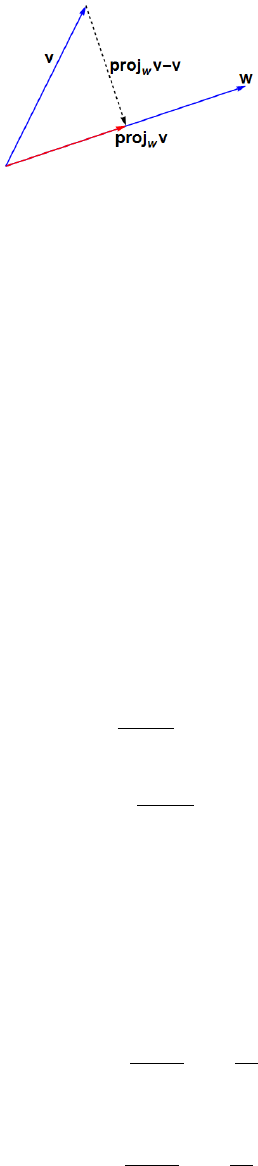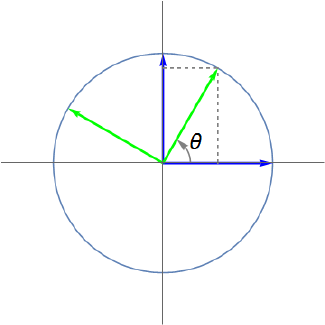Study Guides (400,000)
US (230,000)
UCLA (3,000)
MATH (300)
All (20)
Final

# MATH 33AH Lecture Notes - Lecture 25: Linear Map, Identity Matrix, Unit VectorExam

Department
Mathematics
Course Code
MATH 33AH
Professor
All
Study Guide
Final

This preview shows pages 1-2. to view the full 8 pages of the document.
3 Linear Transformations of the Plane
Now that we’re using matrices to represent linear transformations, we’ll ﬁnd ourselves en-
countering a wide range of transformations and matrices; it can become diﬃcult to keep
track of which transformations do what. In these notes we’ll develop a tool box of basic
transformations which can easily be remembered by their geometric properties.
We’ll focus on linear transformations T:R2R2of the plane to itself, and thus on the
2×2 matrices Acorresponding to these transformation. Perhaps the most important fact
to keep in mind as we determine the matrices corresponding to diﬀerent transformations is
that the ﬁrst and second columns of Aare given by T(e1) and T(e2), respectively, where e1
and e2are the standard unit vectors in R2.
3.1 Scaling
The ﬁrst transformation of R2that we want to consider is that of scaling every vector by
some factor k. That is, T(x) = kxfor every xR2. If k= 1, then Tdoes nothing. In this
case, T(e1) = e1and T(e2) = e2, so the columns of the corresponding matrix Aare e1and
e2:
A=1 0
0 1.
We call this the identity matrix (of size 2) and denote it either as I2or as Iwhen the
size is obvious. For any other scale factor kwe have
T(e1) = k
0and T(e2) = 0
k,
so the corresponding matrix is given by
A=k0
0k.
Now suppose we have two scaling maps: T1which scales by a factor of k1, and T2which
scales by a factor of k2. Then T2T1is the transformation that scales by a factor of k1and
then by k2, which is to say that it scales by a factor of k2k1. This means that its matrix
representation should be given by
A=k2k10
0k2k1,
and indeed we can easily check that
A2A1=k20
0k2k10
0k1=k2k10
0k2k1=A.
This agrees with our notion of matrix multiplication representing composition of linear trans-
formations.
15

Only pages 1-2 are available for preview. Some parts have been intentionally blurred.Figure 2: Orthogonal projection of vonto w.
3.2 Orthogonal Projection
The next linear transformation we’d like to consider is that of projecting vectors onto a line
in R2. First we have to consider what it means to project one vector onto another. Take a
look at Figure 2, where we’re projecting the vector vonto worthogonally. What we mean
by orthogonal projection is that the displacement vector projwvvis orthogonal to the
vector w, as seen in Figure 2.
We can see that projwvwill be a scalar multiple of w, so let’s write projwv=kw. Since
we require that projwvvbe orthogonal to w, we have
(kwv)·w= 0.
That is,
kw·wv·w= 0,
so
k=v·w
w·w.
This means that we have
projwv=v·w
w·ww.
Now notice that if we project vonto any vector which is a nonzero scalar multiple of w, the
resulting vector will be the same as projwv. So really we’re projecting vonto the line L
determined by w. For this reason, we write projLvfor the projection of vonto the line L.
Given a line L, we can compute projLvby ﬁrst selecting a unit vector u=hu1, u2i
through which Lpasses and then projecting vonto u. We then have
projL(e1) = proju(e1) = e1·u
u·uu=u1
1u=u2
1
u1u2
and
projL(e2) = proju(e2) = e2·u
u·uu=u2
1u=u1u2
u2
2,
16

Unlock to view full version

Only pages 1-2 are available for preview. Some parts have been intentionally blurred.Figure 3: Rotation by θ.
so the matrix Acorresponding to the projection onto Lis
A=u2
1u1u2
u1u2u2
2.
As before, there’s a matrix product that’s worth considering here. If we apply the transfor-
mation of projecting onto Ltwice, this should be no diﬀerent than applying it once. After
the ﬁrst projection, all the vectors in R2have been mapped onto L, and projecting a vector
on Lonto Ldoes nothing. This is borne out by the fact that
A2=u2
1u1u2
u1u2u2
2u2
1u1u2
u1u2u2
2=u2
1u1u2
u1u2u2
2=A.
We can also verify our claim that projecting a vector which already lies on Lonto Ldoes
nothing:
Aku1
ku2=ku3
1+ku1u2
2
ku2
1u2+ku3
2=ku1(u2
1+u2
2)
ku2(u2
1+u2
2)=ku1
ku2,
where we using the fact that any vector on Lhas the form hku1, ku2ifor some k.
3.3 Rotation
Next we’ll consider rotating the plane through some angle θ, as depicted in Figure 3. Because
the vector e1lies on the unit circle, so does T(e1), and T(e1) makes an angle of θwith the
x-axis. As a result, its x- and y-components are cos θand sin θ, respectively:
T(e1) = cos θ
sin θ.
At the same time, since e2makes an angle of π/2 with e1, the vectors T(e2) and T(e1)
should also have an angle of π/2 between them. So the x- and y-components of T(e2) are
17